Binomial Probability "At Most" and "At Least" MathBitsNotebook.com Terms of Use   Contact Person: Donna RobertsWhen computing "at least" and "at most" probabilities, it is necessary to consider, in addition to the given probability,      • all probabilities larger than the given probability ("at least")      • all probabilities smaller than the given probability ("at most")
 The probability of an event, p, occurring exactly r times: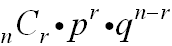n = number of trials  r = number of specific events you wish to obtain  p = probability that the event will occur  q = probability that the event will not occur (q = 1 - p, the complement of the event)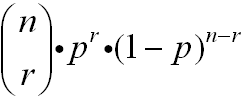Alternative formula formIllustration:
A bag contains 6 red Bingo chips, 4 blue Bingo chips, and 7 white Bingo chips. What is the probability of drawing a red Bingo chip at least 3 out of 5 times? Round answer to the nearest hundredth.

To solve this problem, we need to find the probabilities that r could be 3 or 4 or 5,
to satisfy the condition "at least".
It will be necessary to compute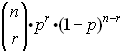for r = 3, r = 4 and r = 5 (which means do the formula three times)
Written in summation notation, we need to compute: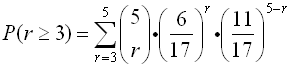For r = 3: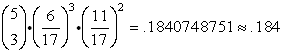For r = 4: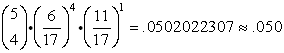For r = 5: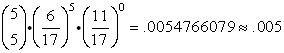Sum: 0.184 + 0.050 + 0.005 = 0.239 rounded to the nearest hundredth = 0.24  ANS

Note: it may be helpful to remember in this problem that
"at most 2 successes" is the compliment of "at least 3 successes".In the following examples, answers will be rounded to the nearest hundredth.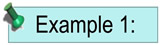A family consists of 3 children.  What is the probability that at most 2 of the children are boys?

Solution:
"At most" 2 boys implies that there could be 0, 1, or 2 boys. The probability of a boy child (or a girl child) is 1/2.

 For r = 0: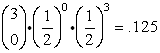For r = 1: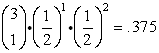For r = 2: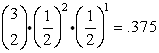Sum: .125 + .375 + .375 = .875 rounded to the nearest hundredth = 0.88  ANS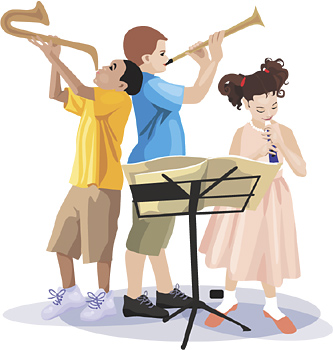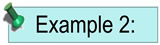Team A and Team B are playing in a league.  They will play each other five times.  If the probability that team A wins a game is 1/3, what is the probability that team A will win at least three of the five games?
Solution:
"At least" 3 wins implies 3, 4, or 5 wins.
 For r = 3: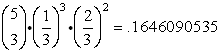For r = 4: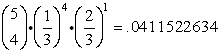For r = 5: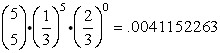Sum: rounded to the nearest hundredth = 0.21  ANS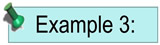As shown in the accompanying diagram, a circular target with a radius of 9 inches has a bull's-eye that has a radius of 3 inches.  If five arrows randomly hit the target, what is the probability that at least four hit the bull's-eye?  Express answer to the nearest thousandth.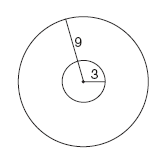Solution:
"At least" 4 hits implies 4 or 5 hits.  The area of the bull's-eye is 9π and the area of the entire target is 81π.  The probability of hitting the desired bull's-eye is 1/9.
 For r = 4: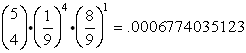For r = 5: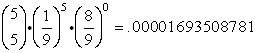Sum: rounded to the nearest thousandth = 0.001  ANS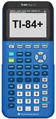For working with Bernoulli Trials on your calculator, click here.For working with At Most At Least on your calculator, click here.